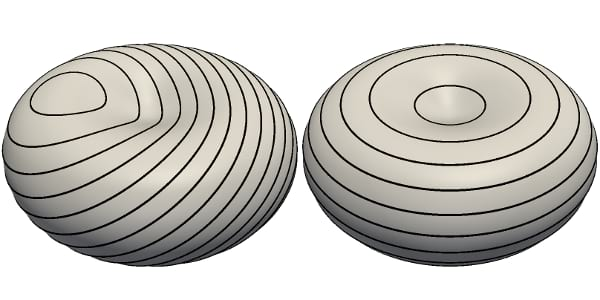## Numerical methods for surface fluidsIn this project we consider a class of surface Navier-Stokes equations which are used to model viscous two-dimensional incompressible fluids on surfaces. We restrict to sufficiently smooth closed oriented (evolving) surfaces embedded in $\mathbb{R}^3$ . For stationary surfaces or evolving surfaces with a prescribed normal velocity the surface Navier-Stokes equations proposed in the literature have different representations but can be shown to be (formally) equivalent. If the normal velocity is not prescribed the derived equations may differ and depend on the underlying physical assumptions. The main topic of the project is the development of efficient and reliable finite element based numerical simulation methods for these surface Navier-Stokes equations. Only very recently, methods that are applicable to general surfaces have been proposed in the literature. We plan to make a systematic comparison and identity important features of these already available techniques. Moreover we address a rigorous stability and error analysis of a class of mixed surface finite element methods. So far, only very few rigorous results for finite element methods applied to surface (Navier-)Stokes problems are known. We expect that in the (near) future this field of simulation methods for surface fluid systems will emerge and many other research groups will become interested in this topic. In view of this we plan to define benchmark problems and provide reliable simulation results for them. Finally, we develop and investigate two model extensions of the surface Navier-Stokes equations, which are inspired by applications studied in the projects Aland/Fischer-Friedrich and Sbalzarini/Voigt, and adapt the considered numerical simulation methods to them.

### Publications

 Maxim A. Olshanskii, Arnold Reusken, and Alexander Zhiliakov, Inf-sup stability of the trace P2-P1 Taylor-Hood elements for surface PDEs, In Mathematics of Computation, Vol. 90, pp. 1527–1555, 2021. [doi] [bibtex] Thomas Jankuhn and Arnold Reusken, Higher order Trace Finite Element Methods for the Surface Stokes Equation, arXiv e-prints, 1909.08327 [math.NA], 2019. [bibtex] Philip Brandner and Arnold Reusken, Finite Element Error Analysis of Surface Stokes Equations in Stream Function Formulation, In ESAIM: M2AN, Vol. 54 (6), pp. 2069–2097, 2020. [doi] [bibtex] Sebastian Reuther, Ingo Nitschke, and Axel Voigt, A numerical approach for fluid deformable surfaces, In Journal of Fluid Mechanics, Vol. 900, pp. R8, 2020. [doi] [bibtex] Thomas Jankuhn, Maxim A. Olshanskii, and Arnold Reusken und Alexander Zhiliakov, Error analysis of higher order Trace Finite Element Methods for the surface Stokes equation, In Journal of Numerical Mathematics, Vol. 29 (3), pp. 245–267, 2021. [doi] [bibtex] Philip Brandner, Arnold Reusken, and Paul Schwering, On derivations of evolving surface Navier–Stokes equations, In Interfaces Free Bound., Vol. 24 (4), pp. 533–563, 2022. [doi] [bibtex] Philip Brandner, Thomas Jankuhn, Simon Praetorius, Arnold Reusken, and Axel Voigt, Finite element discretization methods for velocity-pressure and stream function formulations of surface Stokes equations, In SIAM Journal on Scientific Computing, Vol. 44 (4), 2022. [doi] [bibtex] Arnold Reusken, Analysis of finite element methods for surface vector-Laplace eigenproblems, In Mathematics of Computation, Vol. 91, pp. 1587–1623, 2022. [doi] [bibtex] Maxim A. Olshanskii, Arnold Reusken, and Alexander Zhiliakov, Tangential Navier-Stokes equations on evolving surfaces: Analysis and simulations, In Mathematical Models and Methods in Applied Sciences, Vol. 32 (14), pp. 2817–2852, 2022. [doi] [bibtex]